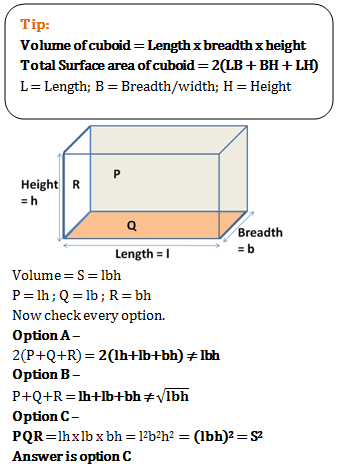# Volume and surface area - Quantitative Aptitude (MCQ) questions for Q. 29492

Q.  Ramesh has a rectangular wooden block. P, Q and R are the areas of the three adjacent and contiguous faces of the block. If we denote its volume by S, then which of the following is true for sure?
- Published on 03 May 17

a. S = 2(P+Q+R)
b. S = P+Q+R
c. S2 = PQR
d. S2 = (PQR)/2